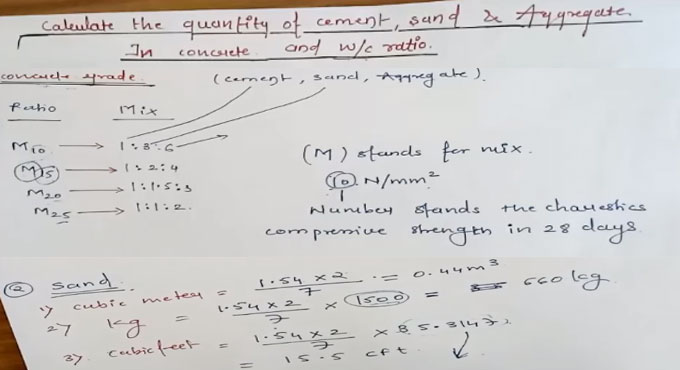# Tips to compute the quantity of cement, sand and aggregate in concrete & water cement ratioIn this construction video tutorial you will learn how to work out the quantity of cement, sand and aggregate in concrete and water cement ratio.

To do it, you should have clear conceptions on the following items :-

M10 - 1:8:6
M15 - 1:2:4
M20 - 1:1.5:3
M25 - 1:1:2

Here, M means Mix and number means the compressive characteristics strength of concrete in 28 days.

Water Cement Ratio :- Grade of cement Water per bag (50 kg) cement (weight of 1 bag cement is 50 kg)

M5 - 60 litre
M7.5 - 45 litre
M10 - 34 litre
M15 - 32 litre
M20 - 30 litre

For calculation purpose, wet volume of concrete = 1 m3

Dry volume = 54% increase by weight volume
Wet volume = 100% + 54% = 154%
In order to covert it to numbers, just divide by 100
154/100 = 1.54

So, dry volume is 1.54 x wet volume
Now, you have to provide the density of cement, fine aggregates and course aggregates as follow :-
Cement = 1440 Kg
Fine Aggregate = 1450 to 1600 Kg/m3
Course Aggregate = 1450 to 1500 Kg/m3

Now, on the basis of the above dimensions, you can start calculation for the different grades of concrete. To know the detailed calculation process, watch the following video tutorial.

Video Source: Parag Pal